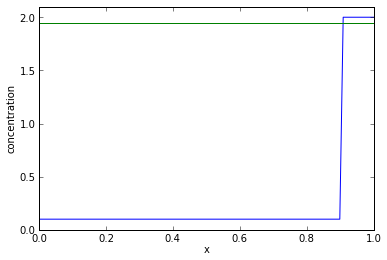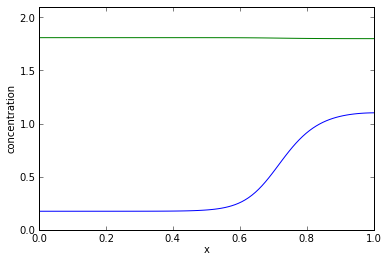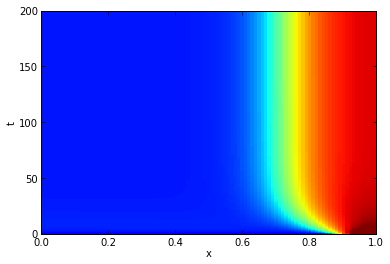This notebook demonstrates how IPython notebooks can be used to discuss the theory and implementation of numerical algorithms on one page.

With ipython nbconvert --to markdown name.ipynb a notebook like this one can be made into a blog post in one easy step. To display the graphics in your resultant blog post use, for instance, your Dropbox Public folder that you can activate here.

# The Crank-Nicolson Method¶

The Crank-Nicolson method is a well-known finite difference method for the numerical integration of the heat equation and closely related partial differential equations.

We often resort to a Crank-Nicolson (CN) scheme when we integrate numerically reaction-diffusion systems in one space dimension

$$\frac{\partial u}{\partial t} = D \frac{\partial^2 u}{\partial x^2} + f(u),$$$$\frac{\partial u}{\partial x}\Bigg|_{x = 0, L} = 0,$$

where $u$ is our concentration variable, $x$ is the space variable, $D$ is the diffusion coefficient of $u$, $f$ is the reaction term, and $L$ is the length of our one-dimensional space domain.

Note that we use Neumann boundary conditions and specify that the solution $u$ has zero space slope at the boundaries, effectively prohibiting entrance or exit of material at the boundaries (no-flux boundary conditions).

## Finite Difference Methods¶

Many fantastic textbooks and tutorials have been written about finite difference methods, for instance a free textbook by Lloyd Trefethen.

Here we describe a few basic aspects of finite difference methods.

The above reaction-diffusion equation describes the time evolution of variable $u(x,t)$ in one space dimension ($u$ is a line concentration). If we knew an analytic expression for $u(x,t)$ then we could plot $u$ in a two-dimensional coordinate system with axes $t$ and $x$.

To approximate $u(x,t)$ numerically we discretize this two-dimensional coordinate system resulting, in the simplest case, in a two-dimensional regular grid. This picture is employed commonly when constructing finite differences methods, see for instance Figure 3.2.1 of Trefethen.

Let us discretize both time and space as follows:

$$t_n = n \Delta t,~ n = 0, \ldots, N-1,$$$$x_j = j \Delta x,~ j = 0, \ldots, J-1,$$

where $N$ and $J$ are the number of discrete time and space points in our grid respectively. $\Delta t$ and $\Delta x$ are the time step and space step respectively and defined as follows:

$$\Delta t = T / N,$$$$\Delta x = L / J,$$

where $T$ is the point in time up to which we will integrate $u$ numerically.

Our ultimate goal is to construct a numerical method that allows us to approximate the unknonwn analytic solution $u(x,t)$ reasonably well in these discrete grid points.

That is we want construct a method that computes values $U(j \Delta x, n \Delta t)$ (note: capital $U$) so that

$$U(j \Delta x, n \Delta t) \approx u(j \Delta x, n \Delta t)$$

As a shorthand we will write $U_j^n = U(j \Delta x, n \Delta t)$ and $(j,n)$ to refer to grid point $(j \Delta x, n \Delta t)$.

## The Crank-Nicolson Stencil¶

Based on the two-dimensional grid we construct we then approximate the operators of our reaction-diffusion system.

For instance, to approximate the time derivative on the left-hand side in grid point $(j,n)$ we use the values of $U$ in two specific grid points:

$$\frac{\partial u}{\partial t}\Bigg|_{x = j \Delta x, t = n \Delta t} \approx \frac{U_j^{n+1} - U_j^n}{\Delta t}.$$

We can think of this scheme as a stencil that we superimpose on our $(x,t)$-grid and this particular stencil is commonly referred to as forward difference.

The spatial part of the Crank-Nicolson stencil (or see Table 3.2.2 of Trefethen) for the heat equation ($u_t = u_{xx}$) approximates the Laplace operator of our equation and takes the following form

$$\frac{\partial^2 u}{\partial x^2}\Bigg|_{x = j \Delta x, t = n \Delta t} \approx \frac{1}{2 \Delta x^2} \left( U_{j+1}^n - 2 U_j^n + U_{j-1}^n + U_{j+1}^{n+1} - 2 U_j^{n+1} + U_{j-1}^{n+1}\right).$$

To approximate $f(u(j \Delta x, n \Delta t))$ we write simply $f(U_j^n)$.

These approximations define the stencil for our numerical method as pictured on Wikipedia.Applying this stencil to grid point $(j,n)$ gives us the following approximation of our reaction-diffusion equation:

$$\frac{U_j^{n+1} - U_j^n}{\Delta t} = \frac{D}{2 \Delta x^2} \left( U_{j+1}^n - 2 U_j^n + U_{j-1}^n + U_{j+1}^{n+1} - 2 U_j^{n+1} + U_{j-1}^{n+1}\right) + f(U_j^n).$$

## Reordering Stencil into Linear System¶

Let us define $\sigma = \frac{D \Delta t}{2 \Delta x^2}$ and reorder the above approximation of our reaction-diffusion equation:

$$-\sigma U_{j-1}^{n+1} + (1+2\sigma) U_j^{n+1} -\sigma U_{j+1}^{n+1} = \sigma U_{j-1}^n + (1-2\sigma) U_j^n + \sigma U_{j+1}^n + \Delta t f(U_j^n).$$

This equation makes sense for space indices $j = 1,\ldots,J-2$ but it does not make sense for indices $j=0$ and $j=J-1$ (on the boundaries):

$$j=0:~-\sigma U_{-1}^{n+1} + (1+2\sigma) U_0^{n+1} -\sigma U_{1}^{n+1} = \sigma U_{-1}^n + (1-2\sigma) U_0^n + \sigma U_{1}^n + \Delta t f(U_0^n),$$$$j=J-1:~-\sigma U_{J-2}^{n+1} + (1+2\sigma) U_{J-1}^{n+1} -\sigma U_{J}^{n+1} = \sigma U_{J-2}^n + (1-2\sigma) U_{J-1}^n + \sigma U_{J}^n + \Delta t f(U_{J-1}^n).$$

The problem here is that the values $U_{-1}^n$ and $U_J^n$ lie outside our grid.

However, we can work out what these values should equal by considering our Neumann boundary condition. Let us discretize our boundary condition at $j=0$ with the backward difference and at $j=J-1$ with the forward difference:

$$\frac{U_1^n - U_0^n}{\Delta x} = 0,$$$$\frac{U_J^n - U_{J-1}^n}{\Delta x} = 0.$$

These two equations make it clear that we need to amend our above numerical approximation for $j=0$ with the identities $U_0^n = U_1^n$ and $U_0^{n+1} = U_1^{n+1}$, and for $j=J-1$ with the identities $U_{J-1}^n = U_J^n$ and $U_{J-1}^{n+1} = U_J^{n+1}$.

Let us reinterpret our numerical approximation of the line concentration of $u$ in a fixed point in time as a vector $\mathbf{U}^n$:

$$\mathbf{U}^n = \begin{bmatrix} U_0^n \\ \vdots \\ U_{J-1}^n \end{bmatrix}.$$

Using this notation we can now write our above approximation for a fixed point in time, $t = n \Delta t$, compactly as a linear system:

$$\begin{bmatrix} 1+\sigma & -\sigma & 0 & 0 & 0 & \cdots & 0 & 0 & 0 & 0\\ -\sigma & 1+2\sigma & -\sigma & 0 & 0 & \cdots & 0 & 0 & 0 & 0 \\ 0 & -\sigma & 1+2\sigma & -\sigma & \cdots & 0 & 0 & 0 & 0 & 0 \\ 0 & 0 & \ddots & \ddots & \ddots & \ddots & 0 & 0 & 0 & 0 \\ 0 & 0 & 0 & 0 & 0 & 0 & 0 & -\sigma & 1+2\sigma & -\sigma \\ 0 & 0 & 0 & 0 & 0 & 0 & 0 & 0 & -\sigma & 1+\sigma \end{bmatrix} \begin{bmatrix} U_0^{n+1} \\ U_1^{n+1} \\ U_2^{n+1} \\ \vdots \\ U_{J-2}^{n+1} \\ U_{J-1}^{n+1} \end{bmatrix} = \begin{bmatrix} 1-\sigma & \sigma & 0 & 0 & 0 & \cdots & 0 & 0 & 0 & 0\\ \sigma & 1-2\sigma & \sigma & 0 & 0 & \cdots & 0 & 0 & 0 & 0 \\ 0 & \sigma & 1-2\sigma & \sigma & \cdots & 0 & 0 & 0 & 0 & 0 \\ 0 & 0 & \ddots & \ddots & \ddots & \ddots & 0 & 0 & 0 & 0 \\ 0 & 0 & 0 & 0 & 0 & 0 & 0 & \sigma & 1-2\sigma & \sigma \\ 0 & 0 & 0 & 0 & 0 & 0 & 0 & 0 & \sigma & 1-\sigma \end{bmatrix} \begin{bmatrix} U_0^{n} \\ U_1^{n} \\ U_2^{n} \\ \vdots \\ U_{J-2}^{n} \\ U_{J-1}^{n} \end{bmatrix} + \begin{bmatrix} \Delta t f(U_0^n) \\ \Delta t f(U_1^n) \\ \Delta t f(U_2^n) \\ \vdots \\ \Delta t f(U_{J-2}^n) \\ \Delta t f(U_{J-1}^n) \end{bmatrix}.$$

Note that since our numerical integration starts with a well-defined initial condition at $n=0$, $\mathbf{U}^0$, the vector $\mathbf{U}^{n+1}$ on the left-hand side is the only unknown in this system of linear equations.

Thus, to integrate numerically our reaction-diffusion system from time point $n$ to $n+1$ we need to solve numerically for vector $\mathbf{U}^{n+1}$.

Let us call the matrix on the left-hand side $A$, the one on the right-hand side $B$, and the vector on the right-hand side $\mathbf{f}^n$. Using this notation we can write the above system as

$$A \mathbf{U}^{n+1} = B \mathbf{U}^n + f^n.$$

In this linear equation, matrices $A$ and $B$ are defined by our problem: we need to specify these matrices once for our problem and incorporate our boundary conditions in them. Vector $\mathbf{f}^n$ is a function of $\mathbf{U}^n$ and so needs to be reevaluated in every time point $n$. We also need to carry out one matrix-vector multiplication every time point, $B \mathbf{U}^n$, and one vector-vector addition, $B \mathbf{U}^n + f^n$.

The most expensive numerical operation is inversion of matrix $A$ to solve for $\mathbf{U}^{n+1}$, however we may get away with doing this only once and store the inverse of $A$ as $A^{-1}$:

$$\mathbf{U}^{n+1} = A^{-1} \left( B \mathbf{U}^n + f^n \right).$$

## A Crank-Nicolson Example in Python¶

Let us apply the CN method to a two-variable reaction-diffusion system that was introduced by Mori et al.:

$$\frac{\partial u}{\partial t} = D_u \frac{\partial^2 u}{\partial x^2} + f(u,v),$$$$\frac{\partial v}{\partial t} = D_v \frac{\partial^2 v}{\partial x^2} - f(u,v),$$

with Neumann boundary conditions

$$\frac{\partial u}{\partial x}\Bigg|_{x=0,L} = 0,$$$$\frac{\partial v}{\partial x}\Bigg|_{x=0,L} = 0.$$

The variables of this system, $u$ and $v$, represent the concetrations of the active form and its inactive form respectively. The reaction term $f(u,v)$ describes the interchange (activation and inactivation) between these two states of the protein. A particular property of this system is that the inactive has much greater diffusivity that the active form, $D_v \gg D_u$.

Using the CN method to integrate this system numerically, we need to set up two separate approximations

$$A_u \mathbf{U}^{n+1} = B_u \mathbf{U}^n + \mathbf{f}^n,$$$$A_v \mathbf{V}^{n+1} = B_v \mathbf{V}^n - \mathbf{f}^n,$$

with two different $\sigma$ terms, $\sigma_u = \frac{D_u \Delta t}{2 \Delta x^2}$ and $\sigma_v = \frac{D_v \Delta t}{2 \Delta x^2}$.

### Import Packages¶

For the matrix-vector multiplication, vector-vector addition, and matrix inversion that we will need to carry out we will use the Python library NumPy. To visualize our numerical solutions, we will use pyplot.

In :
import numpy
from matplotlib import pyplot


Numpy allows us to truncate the numerical values of matrices and vectors to improve their display with set_printoptions.

In :
numpy.set_printoptions(precision=3)


### Specify Grid¶

Our one-dimensional domain has unit length and we define J = 100 equally spaced grid points in this domain. This divides our domain into J-1 subintervals, each of length dx.

In :
L = 1.
J = 100
dx = float(L)/float(J-1)
x_grid = numpy.array([j*dx for j in range(J)])


Equally, we define N = 1000 equally spaced grid points on our time domain of length T = 200 thus dividing our time domain into N-1 intervals of length dt.

In :
T = 200
N = 1000
dt = float(T)/float(N-1)
t_grid = numpy.array([n*dt for n in range(N)])


### Specify System Parameters and the Reaction Term¶

We choose our parameter values based on the work by Mori et al..

In :
D_v = float(10.)/float(100.)
D_u = 0.01 * D_v

k0 = 0.067
f = lambda u, v: dt*(v*(k0 + float(u*u)/float(1. + u*u)) - u)
g = lambda u, v: -f(u,v)

sigma_u = float(D_u*dt)/float((2.*dx*dx))
sigma_v = float(D_v*dt)/float((2.*dx*dx))

total_protein = 2.26


### Specify the Initial Condition¶

As discussed by Mori et al., we can expect to observe interesting behaviour in the steady state of this system if we choose a heterogeneous initial condition for $u$.

Here, we initialize $u$ with a step-like heterogeneity:

In :
no_high = 10
U =  numpy.array([0.1 for i in range(no_high,J)] + [2. for i in range(0,no_high)])
V = numpy.array([float(total_protein-dx*sum(u))/float(J*dx) for i in range(0,J)])


Note that we make certain that total protein amounts equal a certain value, total_protein. The importance of this was discussed by Walther et al..

Let us plot our initial condition for confirmation:

In :
ylim((0., 2.1))
xlabel('x'); ylabel('concentration')
pyplot.plot(x_grid, U)
pyplot.plot(x_grid, V)
pyplot.show()The blue curve is the initial condition for $U$, stored in Python variable U, and the green curve is the initial condition for $V$ stored in V.

### Create Matrices¶

The matrices that we need to construct are all tridiagonal so they are easy to construct with numpy.diagflat.

In :
A_u = numpy.diagflat([-sigma_u for i in range(J-1)], -1) +\
numpy.diagflat([1.+sigma_u]+[1.+2.*sigma_u for i in range(J-2)]+[1.+sigma_u]) +\
numpy.diagflat([-sigma_u for i in range(J-1)], 1)

B_u = numpy.diagflat([sigma_u for i in range(J-1)], -1) +\
numpy.diagflat([1.-sigma_u]+[1.-2.*sigma_u for i in range(J-2)]+[1.-sigma_u]) +\
numpy.diagflat([sigma_u for i in range(J-1)], 1)

A_v = numpy.diagflat([-sigma_v for i in range(J-1)], -1) +\
numpy.diagflat([1.+sigma_v]+[1.+2.*sigma_v for i in range(J-2)]+[1.+sigma_v]) +\
numpy.diagflat([-sigma_v for i in range(J-1)], 1)

B_v = numpy.diagflat([sigma_v for i in range(J-1)], -1) +\
numpy.diagflat([1.-sigma_v]+[1.-2.*sigma_v for i in range(J-2)]+[1.-sigma_v]) +\
numpy.diagflat([sigma_v for i in range(J-1)], 1)


To confirm, this is what A_u looks like:

In :
print A_u

[[ 1.981 -0.981  0.    ...,  0.     0.     0.   ]
[-0.981  2.962 -0.981 ...,  0.     0.     0.   ]
[ 0.    -0.981  2.962 ...,  0.     0.     0.   ]
...,
[ 0.     0.     0.    ...,  2.962 -0.981  0.   ]
[ 0.     0.     0.    ..., -0.981  2.962 -0.981]
[ 0.     0.     0.    ...,  0.    -0.981  1.981]]


### Solve the System Iteratively¶

To advance our system by one time step, we need to do one matrix-vector multiplication followed by one vector-vector addition on the right hand side.

To facilitate this, we rewrite our reaction term so that it accepts concentration vectors $\mathbf{U}^n$ and $\mathbf{V}^n$ as arguments and returns vector $\mathbf{f}^n$.

As a reminder, this is our non-vectorial definition of $f$

f = lambda u, v: v*(k0 + float(u*u)/float(1. + u*u)) - u
In :
f_vec = lambda U, V: numpy.multiply(dt, numpy.subtract(numpy.multiply(V,


Let us make certain that this produces the same values as our non-vectorial f:

In :
print f(U, V)

0.00996135898275

In :
print f(U[-1], V[-1])

-0.0623832232232

In :
print f_vec(U, V)

[ 0.01   0.01   0.01   0.01   0.01   0.01   0.01   0.01   0.01   0.01   0.01
0.01   0.01   0.01   0.01   0.01   0.01   0.01   0.01   0.01   0.01   0.01
0.01   0.01   0.01   0.01   0.01   0.01   0.01   0.01   0.01   0.01   0.01
0.01   0.01   0.01   0.01   0.01   0.01   0.01   0.01   0.01   0.01   0.01
0.01   0.01   0.01   0.01   0.01   0.01   0.01   0.01   0.01   0.01   0.01
0.01   0.01   0.01   0.01   0.01   0.01   0.01   0.01   0.01   0.01   0.01
0.01   0.01   0.01   0.01   0.01   0.01   0.01   0.01   0.01   0.01   0.01
0.01   0.01   0.01   0.01   0.01   0.01   0.01   0.01   0.01   0.01   0.01
0.01   0.01  -0.062 -0.062 -0.062 -0.062 -0.062 -0.062 -0.062 -0.062
-0.062 -0.062]


Accounting for rounding of the displayed values due to the set_printoptions we set above, we can see that f and f_vec generate the same values for our initial condition at both ends of our domain.

We will use numpy.linalg.solve to solve our linear system each time step.

While we integrate our system over time we will record both U and V at each time step in U_record and V_record respectively so that we can plot our numerical solutions over time.

In :
U_record = []
V_record = []

U_record.append(U)
V_record.append(V)

for ti in range(1,N):
U_new = numpy.linalg.solve(A_u, B_u.dot(U) + f_vec(U,V))
V_new = numpy.linalg.solve(A_v, B_v.dot(V) - f_vec(U,V))

U = U_new
V = V_new

U_record.append(U)
V_record.append(V)


### Plot the Numerical Solution¶

Let us take a look at the numerical solution we attain after N time steps.

In :
ylim((0., 2.1))
xlabel('x'); ylabel('concentration')
pyplot.plot(x_grid, U)
pyplot.plot(x_grid, V)
pyplot.show()And here is a kymograph of the values of U. This plot shows concisely the behaviour of U over time and we can clear observe the wave-pinning behaviour described by Mori et al.. Furthermore, we observe that this wave pattern is stable for about 50 units of time and we therefore conclude that this wave pattern is a stable steady state of our system.

In :
U_record = numpy.array(U_record)
V_record = numpy.array(V_record)

fig, ax = subplots()
xlabel('x'); ylabel('t')
heatmap = ax.pcolor(x_grid, t_grid, U_record, vmin=0., vmax=1.2)